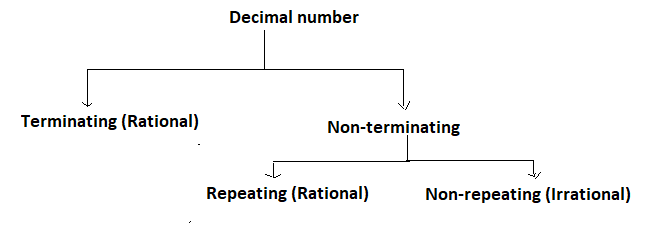QuestionAnswers

# Classify the following number as rational or irrational. 30.2323422345…Verified
113.1K+ Views
Hint: To check whether the decimal number is rational or irritation first, we have to check whether it is terminating or not. And if it is non-terminating then check whether its digits are repeating or not. And if digits are not repeating then it will be irrational.

Now as we know that according to the definition of rational number any number is known as rational number if it is expressed as quotient or fraction $\dfrac{p}{q}$ of two integers where p is the numerator and q is the denominator. Since p and q are integers. So, q can also be equal to 1. So, every integer is a rational number.
Like $2,{\text{ }} - 5,{\text{ }}0,{\text{ }}\dfrac{{ - 6}}{5},{\text{ }} - \dfrac{{71}}{9}$ are some of the rational numbers.
And according to the definition of irrational number any number is known as irrational if it is not rational or in other words we can say that the number cannot expressed as quotient or fraction $\dfrac{p}{q}$ of two integers where p is the numerator and q is the denominator and both are integers.
Like $\pi ,{\text{ }}0.0001010011010....$ are some of the irrational numbers.